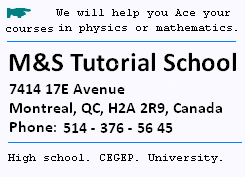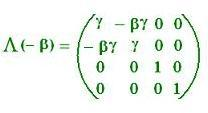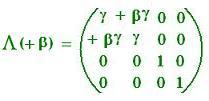Contents# Relativity: The 4-Vectors

### 1. Lorentz Transformations

From the reference frame at rest (RF):
The velocity of the reference frame (RF) is 0
The velocity of the moving reference frame (MF) is v
The velocity of the moving object is u.

From the moving frame (MF):
The velocity of the moving reference frame (MF) is 0
The velocity of the reference frame (RF) is - v
The velocity of the moving object is u'

In the (MF):
Galilean transformations give:

t' = t
x' = x - vt
y' = y
z' = z

and
Lorentz Transformations give:

t' = γ(t - vx/c2)
x' = γ(x - vt)
y' = y
z' = z

where
γ = 1/[1 - β2]1/2, and β = v/c

We have also the reverse transformation:

t = γ(t' + vx'/c2)
x = γ(x' + vt')
y = y'
z = z'

### 2. Proper time

If an object is at rest in the mooving reference frame u' = 0. So its velocity in the (RF) is v, because the velocity of the (MF) is v in the (RF).

In the (RF):
If the object starts to move at t = 0 along the x-axis. At a time later t, the object is located at:
x = vt, y = 0, z = 0, t = t

In the (MF):
The object is located at:
x' = γ(vt - vt) = 0
y' = y
z' = z
t' = γ(t - v vt /c2) =
γ t (1 - (v /c)2) = γ t (1/γ2) = t/γ

In the (RF): time = t, and
In the (MF): time = t' = t/γ < t
In the (RF) t = γ t' : The time is dilated.

t' is the proper time, which measures time in the moving reference frame.

### 3. 4-Vectors notations

For an event E = (t, x, y, z), Lorentz transformation is then an operator that transforms this 4-Vector on another 4-Vector event E' = (t', x', y', z') = (γ(t - vx/c2), γ(x - vt), y, z) within a certain vector space called the Minkowski space, which is a 4-Dimension Cartesian space with a specific property of the inner product.

But, in order to have the same dimension, that is a length, for all of the 4 components, we just make following change in Lorentz transformations, and get:

ct' = γ(ct - vxc/c2) x' = γ(x - vtc/c)
y' = y
z' = z

That is:
ct' = γ(ct - βx) x' = γ(x - β ct)
y' = y
z' = z

E = (ct, x, y, z), transformed in:
E' = (ct', x', y', z') = (γ(ct - βx), γ(x - β ct), y, z)

### 4. The invariant (Δs)2 = - c2t2 + r2

In the (MF):The event E = (ct, x, y, z), transformed into the event:
E' = (ct', x', y', z') = (γ(ct - βx), γ(x - β ct), y, z),

ct' = γ(ct - βx) x' = γ(x - β ct)
y' = y
z' = z

Therefore
E' = Λ(- β) E

In the (RF):The event E' = (ct', x', y', z'), is transformed in the event:
E = (ct, x, y, z) = (γ(ct' + βx'), γ(x' + βct'), y', z')

ct = γ(ct' + βx') x = γ(x' + β ct')
y = y'
z = z'

Therefore

E = Λ(+β) E'

That we can write:
c(-t') = γ(c(-t') - βx')
x = γ(x' - β c (-t'))
y = y'
z = z'

So, in the (MF):
The event E' = (-ct', x', y', z'), is transformed in the event:
E = (-ct, x, y, z) = (γ(-ct' - βx'), γ(x' - βct'), y', z')

Therefore

E = Λ(-β) E'

That is:

If E is tranformed with Λ(-β) in E', E' is tranforme with Λ(+β) in E. Thus, to transform E' in E with Λ(-β), we have to change the time t' into -t'. That is we replace (ct, x, y, z) = Λ(+β) (ct', x', y', z') by (-ct, x, y, z) = Λ(- β) (-ct', x', y', z')

Hence:
(ct', x', y', z') = Λ(-β) (ct, x, y, z)
(-ct, x, y, z) = Λ(- β) (-ct', x', y', z')

or

(ct, x, y, z) = Λ- 1(ct', x', y', z')
(-ct, x, y, z) = Λ(- β) (-ct', x', y', z')

The scalar product gives:
(ct, x, y, z) . (-ct, x, y, z) = Λ- 1(ct', x', y', z') . Λ(- β) (-ct', x', y', z') = (ct', x', y', z') (-ct', x', y', z')

or
(ct, x, y, z) . (-ct, x, y, z) = (ct', x', y', z') (-ct', x', y', z')

That is
- c2t2 + x2 + y2 + z2 = - c2t'2 + x'2 + y'2 + z'2

Therefore, if the vector space r has the components x, y , and z:

Under Lorentz transormations, the squared norm of an event is constant: The squared distance: - c2t2 + x2 + y2 + z2 = - c2t2 + r2 is an invariant .

(Δs)2 = - c2t2 + r2 is invariant .

### 5. Covariance and contravariance Notations

We will use the following notation:

For the kind of 4-Vector: (ct, x, y, z):
(ct, x, y, z) = (x0, x1, x2, x3) or xμ for short, μ = 0, 1, 2, 3.

xμ is called contravariant 4-vector.

For the kind of 4-Vector: (- ct, x, y, z):
(- ct, x, y, z) = (x0, x1, x2, x3) or xμ for short, μ = 0, 1, 2, 3.

xμ is called covariant 4-vector.

So

(Δs)2 = xμ . xμ = Σ xμ xμ
μ = 0, 1, 2, 3.
= x0x0 + x1x1 + x2x2 + x3x3

The fact that to use xμ for short, leads to following notation called Einstein notation:
Σ xμ xμ = xμ xμ
μ = 0, 1, 2, 3.

Let's find again that the defined scalar product is invariant:
(a0, a) = aμ un event, Lorentz-transformed into (a'0, a') = a'μ, and
(b0, b) = bμ un event, Lorentz-transformed into (b'0, b') = b'μ

Remark that
bμ = (b0, b) = (- b0, b)

a'μb'μ = (a'0, a')(b'0, b') = - γ(a0 - βa1)γ(b0 - βb1) + γ(a1 - β a0)γ(b1 - β b0) + a2b2 + a3b3 =

γ2[ - a0b0 + βa1b0 βa0b1 + βb1a0 - βb1βa1 + a1b1 - β a0b1 - β b0a1 + β b0β a0] + a2b2 + a3b3

= γ2[ (a0b0 - a1b1) (β2 - 1)] a2b2 + a3b3 =

- a0b0 + a1b1 a2b2 + a3b3

= aμbμ

Therefore

a'μb'μ = aμbμ = invariant

Remark:
aμbμ = (a0, a)(b0, b) =
(a0, a)(- b0, b) = (- a0, a)(b0, b) =
= ( a0, a)(b0, b) = aμbμ

Therefore

aμbμ = aμbμ

 chimie labs | Physics and Measurements | Probability & Statistics | Combinatorics - Probability | Chimie | Optics | contact |